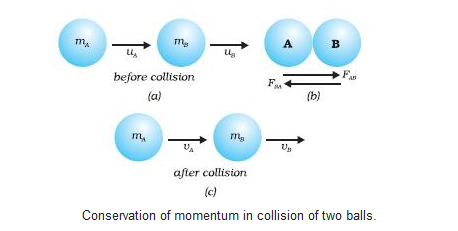# Class 9 Force and Laws of Motion Notes

Force and Laws of Motion Notes

## Force:-

A push or pull on an object is called force.

## A force can change-

• the state of motion of an object i.e, motion to rest or, rest to motion.
• the speed of the object
• the direction of motion
• the shape of the object

## Contact Force:-

The force exerted by one object on another object due to the physical contact between them is called contact force.

Ex:- muscular force, frictional force etc.

## Non-contact Force:-

The force exerted by one object on another object without any physical contact between them is called non-contact force.

Ex:- Magnetic force, electrostatic force, gravitational force etc.

## Balanced Forces:-

If the net force acting on an object is zero, then the forces acting on the object are said to be balanced.

## Unbalanced Forces:-

If the net force acting on an object is not zero, then the forces acting on the object are said to be unbalanced.

## Newton’s First Law of Motion (Law of Inertia):-

An object remains in a state of rest or of uniform motion in a straight line unless or until an unbalanced force is applied.

Or, All objects resist a change in their state of motion.

### Inertia:-

The natural tendency of an object to resist a change in its state of motion or of rest is called inertia.

#### Inertia is classified as:-

• Inertia of rest: Ex – A person seating in a car leans backward when the car starts all of a sudden
• Inertia of motion: Ex – A person seating in a moving car leans forward when the car stops all of a sudden
• Inertia of direction: Ex – Whenever a bus takes a sharp turn, the passengers experience a force acting away from the centre of the curve
• Mass of an object is a measure of its inertia.
• Inertia ∝ Mass

### Momentum:-

The momentum of an object is defined as the product of its mass and velocity.

• p = mv
• S.I. unit:- kg m/s

## Newton’s Second Law of Motion:-

The force applied on an object is directly proportional to the rate of change of momentum in the direction of force.

### Mathematical Formulation of Second Law of Motion:-

Consider an object of mass ‘m’ moving in a straight line with initial velocity ‘u’. It is uniformly accelerated to final velocity ‘v’ in time ‘t’.

So, initial momentum = p1 = mu and final momentum = p2 =mv

A/c to second law of motion,

Force applied ∝ Change in momentum/time

or, F ∝ (p2 – p1)/t

or, F ∝ (mv – mu)/t

or, F ∝ m(v-u)/t

or, F ∝ ma [since, a = (v-u)/t]

or, F = k m a where, k is constant of proportionality

Here, k = 1 So, F = ma

• S.I. unit of force:- kg m/s2 or Newton(N)
• 1 Newton = 1 kg m/s2 = 105 Dyne
• 1 Newton of force:- The amount of force is said to be 1 newton that produces an acceleration of 1 m/s2 in an object of 1 kg mass.

### Proof of First Law of Motion Using Second Law of Motion:-

From second law of motion,

F = ma

or, F = m(v-u)/t

or, Ft/m = v-u

when, F = 0

then, v-u = 0

or, v = u

Here, we see that when external applied force is 0, then there is no change in its state of motion or of rest which is the first law of motion.

## Newton’s Third Law of Motion:-

It states that every action has equal and opposite reaction.

Ex:- A forward force on the bullet and recoil of the gun.

## Law of Conservation of Momentum:-

The sum of momenta of the two objects before collision is equal to the sum of momenta after the collision provided there is no external unbalanced force acting on them.

### Mathematical Proof of Law of Conservation of Momentum:-

Consider two objects having masses mA and mB moving with initial velocities uA and uB respectively in the same direction such that uA > uB. After collision, let their final velocities be vA and vB respectively.

.From 2nd law of motion,

Force exerted by A on B = FAB = mA(vA-uA)/t

Force exerted by B on A = FBA = mB(vB-uB)/t

From 3rd law of motion,

FAB = -FBA

or, mA(vA-uA)/t = -mB(vB-uB)/t

or, mAvA – mAuA = -mBvB + mBuB

or, mAuA + mBuB = mAvA + mBvB